## Saturday, September 26, 2020

### DOFIS: The start of a 'major' update

As mentioned in previous posts, the current version of DOFIS assumes a symmetrical lens, albeit a split, thin lens. In a symmetric lens, the entrance and exit pupils are collocated at the front and rear principal planes.

Ignoring the lens asymmetry is OK for most photography, other than macro work and those interested in knowing the position of the entrance pupil, ie the pano pivot point.

I've therefore decided to update the (registered) lens model in DOFIS, and account for asymmetry: that is the pupillary magnification (p = exit-pupil/entrance-pupil).

This post is directed at creating the model, that I will be coding up in DOFIS.

It is easy to see if you have a non-symmetric lens, by simply looking at the lens from the back and the front, where you see the exit and the entrance pupils.

As an example, on the left we see a near symmetric lenses and on the right a retro-focus lens, where the exit pupil is larger than the entrance pupil. Most/all wide angle lenses are of a retro focus design.

Measuring the pupil magnification (p) is relatively easy, eg focus on the pupil and put a ruler next to the lens, or better put the ruler in the ‘same’ plane as the pupil. and take an in focus image of the pupils, ie from the rear and front.

Now scale the images to match each ruler and measure the ratio between the exit and entrance diameters.

In a previous post I showed how to measure the magnification (m) of the lens, ie relative to the sensor, in order to estimate the lens thickness, or hiatus; once again, you will need to do this at the widest and longest focal lengths of a zoom.

For a zoom lens, knowing/measuring m and p at the two focal length extremes, we will assume a linear relationship in between.

Let's now remind ourselves of what we are trying to model; for example a Sigma 12-24mm F4 DG HSM, with its 11 groups and 17 lens elements: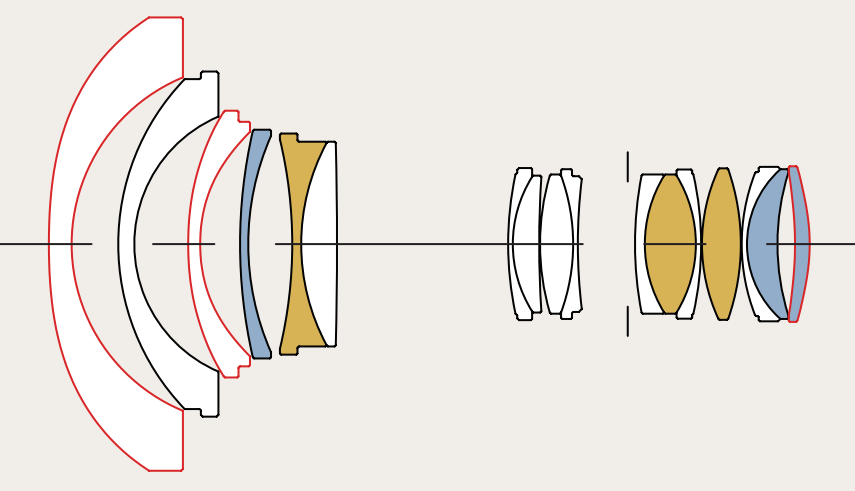As can be seen, the lens layout is rather complicated, plus we have no real info on the lens design, eg position of the front principal etc. So we need another way to handle things.

It is well know that any lens may be treated as a 'black box' by knowing the focal length and the position of four cardinal 'planes' along the lens axis:

• The front principal (H)
• The rear principal (H')
• The entrance pupil (E)
• The exit pupil (X)

Knowing the above allows us to 'map' light rays entering from the real world, being projected onto the sensor: all without knowing the actual lens design. They also allow us to correctly find the field of view and pano pivot point as the lens changes focus, ie we are not interested in cine lenses here, but photographic lenses.

We now have this, where 'all' we need to do is work out the positions: E, H, H' and X: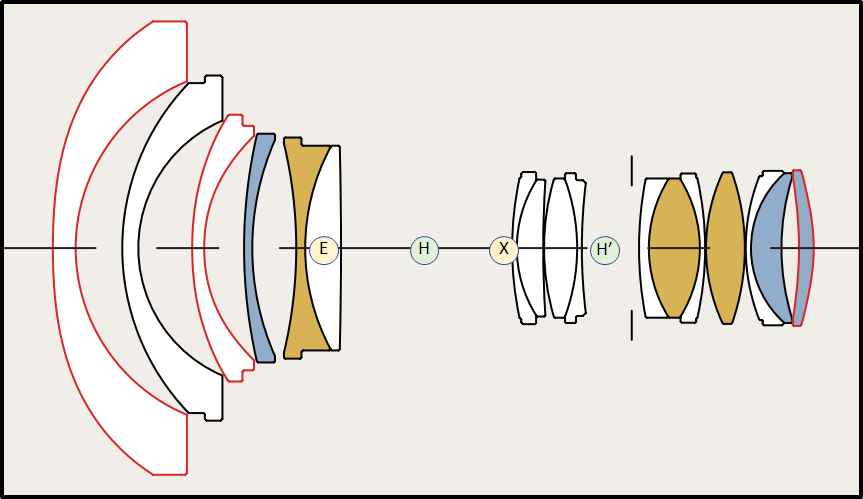Note the positions of E, H, X and H' above are illustrative: they can move relative to each other in radical ways according to the lens design and the focal length, eg retro focus etc.

We now need to add in a camera body and a sensor at the image plane (I):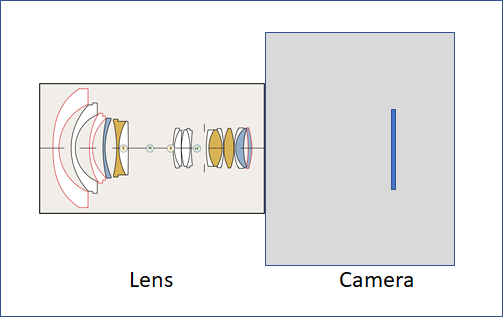Plus, let's not forget a subject, positioned at the object plane (O):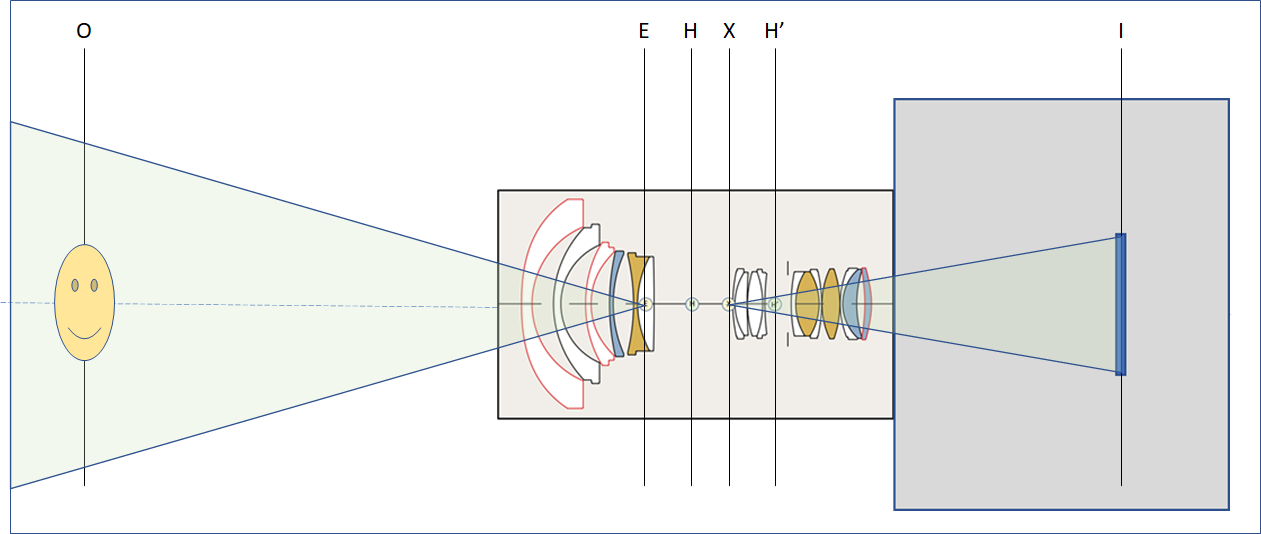A key point to note in the above is the location of the apex of the field of view (FoV), and its projection on the sensor. That is the pupil planes and not the front/rear principal planes.

All the equations we now need may be derived from the lens makers equation and simple optical geometry. For those that wish to understand more I can recommend reading D. Andrew Rowlands Field Guide to Photographic Science: https://spie.org/Publications/Book/2542354?&origin_id=x31902. Another good read on this subject can be found at https://www.panohelp.com/thinlensformula.html

We can now dispense with the complications of a 'real lens' and just look at the planes of interest. Note the introduction of the, so-called, hiatus, between H and H’, which we call the split lens thickness (t) in this model. The following layout is for where p > 1, ie indicating a retro focus lens; if p < 1 the exit and entrance pupils move to the other side of the principal planes.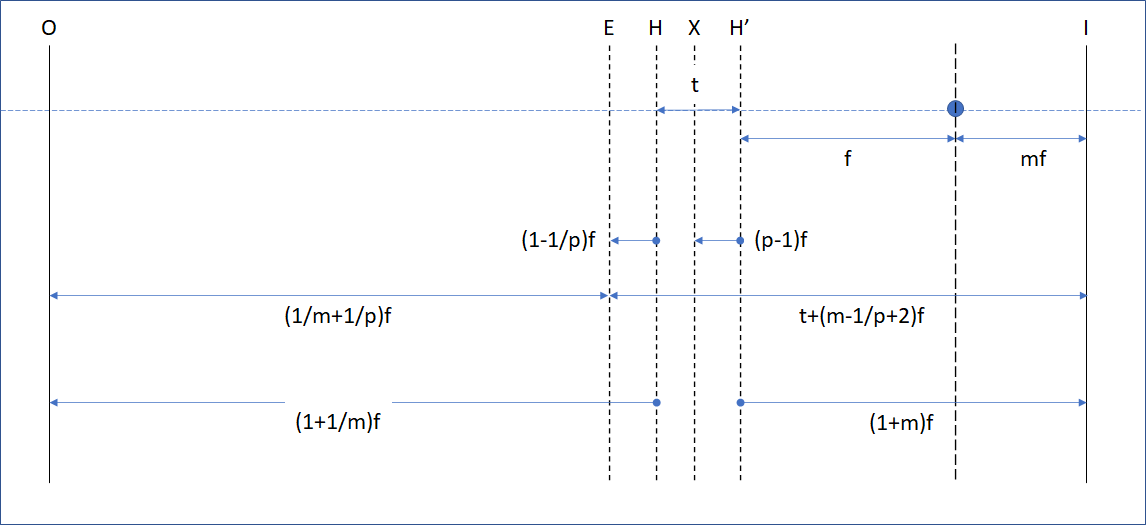To complete the model let's add in the near and far depths of field: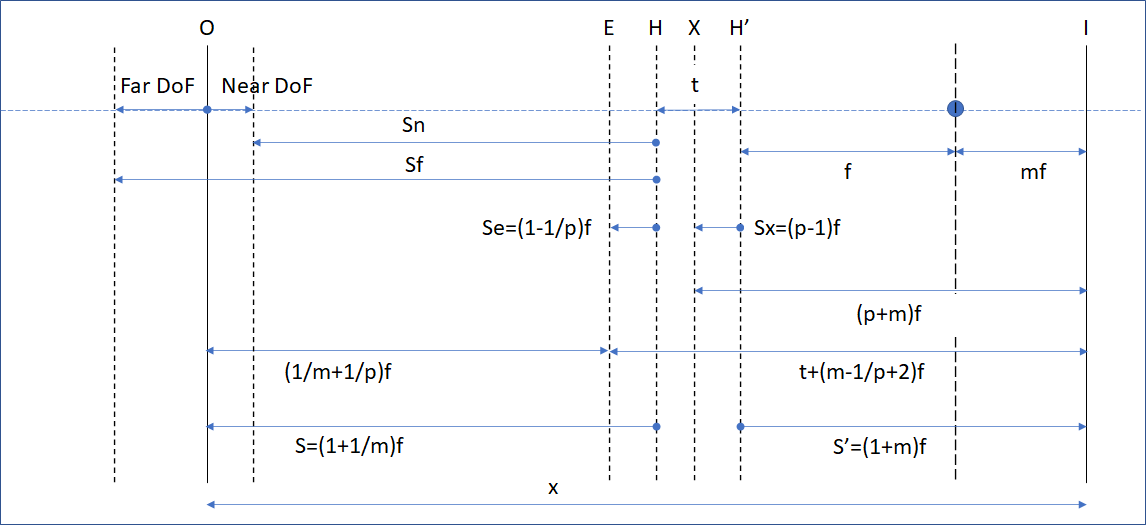From the above we can calculate the near and far depths of field, simply by knowing: the distance from the sensor to the object in focus (x), which Magic Lantern gives us from Canon; the focal length (f); the reproduction ratio, or magnification, (m); the pupil ratio (p), ie exit/entrance pupil sizes; the aperture (N); and the CoC (C).

To help us on our way, let's first capture the hyperfocal, H:

Here we see the impact of the pupillary magnification (p), which is normally ignored, ie assumed to be unity, giving us the 'usual' form of the hyperfocal, ie h + f. For landscapes etc, h on its own is a pretty good approximation for the hyperfocal: noting, of course, that the hyperfocal, like the FoV, is not measured from the sensor, but from the entrance pupil (E).

We need a way to move from the sensor to object space, to the front principal to object space. This is achieved via the following simple relationship: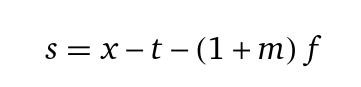From a previous post we know how to calculate the lens thickness at an given focal length: http://photography.grayheron.net/2020/08/pragmatism-not-perfection.html

As for m, which varies as focus varies, we can use the following equation, where q=x-t:

We now have everything we need to calculate such things as the near and far depths of field.

With the far DoF being:
Where e is Se in the diagram above.

In future posts I'll present other details on the model and, finally, introduce the DOFIS uplift, which will include the following additional functionality over the current DOFIS version:

• Account for lens asymmetry;
• Provide an estimated position of the entrance pupil relative to the sensor plane, ie the pano pivot location;
• Some additional info that photographers should find of value.
As usual I welcome any feedback on this post.